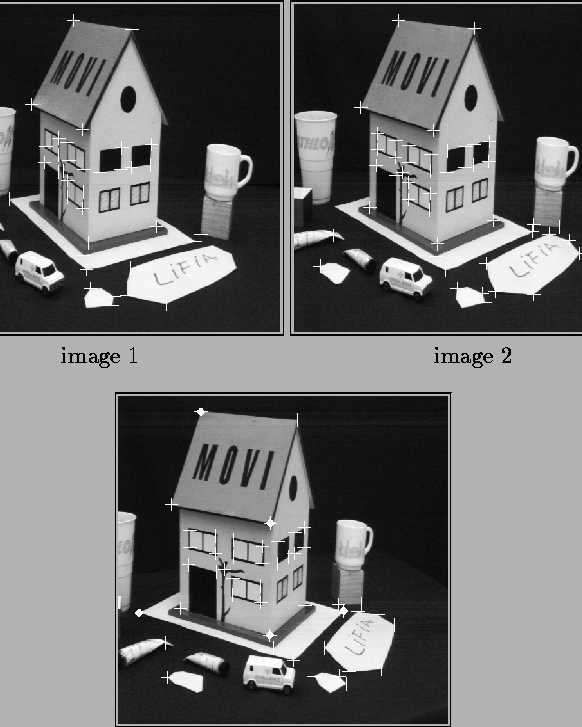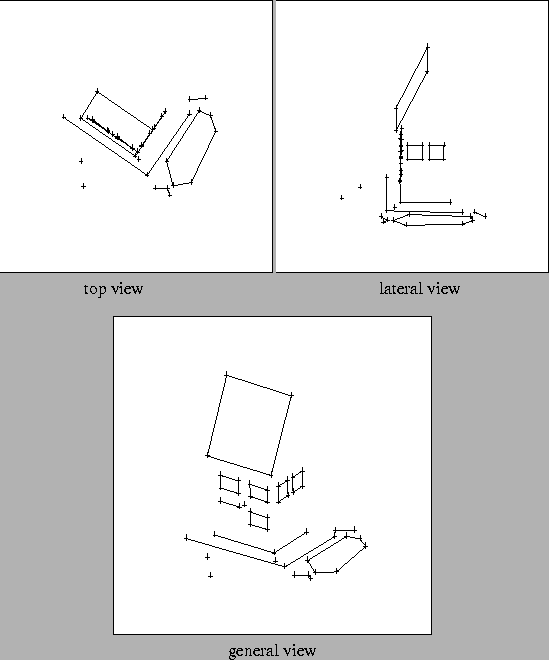Next: Self Calibration Up: 3D Reconstruction from Multiple Previous: Affine Reconstruction

## Euclidean Reconstruction

We have not implemented the specific suggestions of section 4.2 for the recovery of Euclidean structure from a projective reconstruction by observing known scene angles, circles,...However we have developed a more brute force approach, finding a projective transformation H in equation (5.4) that maps the projective reconstruction to one that satisfies a set of redundant Euclidean constraints. This simultaneously minimizes the error in the projection equations and the violation of the constraints. The equations are highly nonlinear and a good initial guess for the structure is needed. In our experiments this was obtained by assuming parallel projection, which is linear and allows easy reconstruction using SVD decomposition ().

Figures 5.3 and 5.4 show an example of this process.Next: Self Calibration Up: 3D Reconstruction from Multiple Previous: Affine Reconstruction
Bill Triggs
1998-11-13